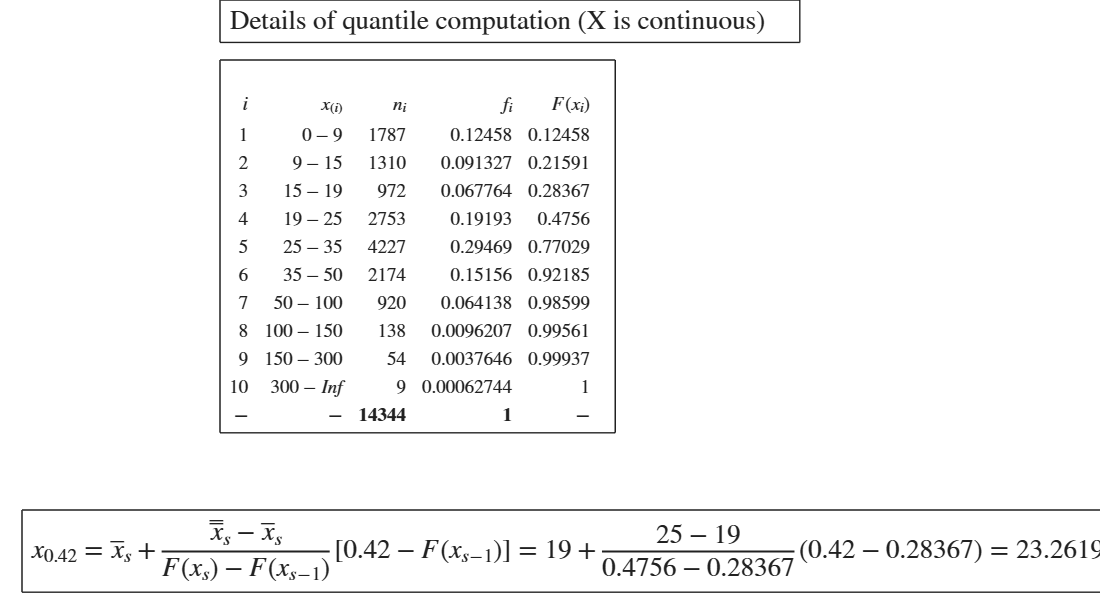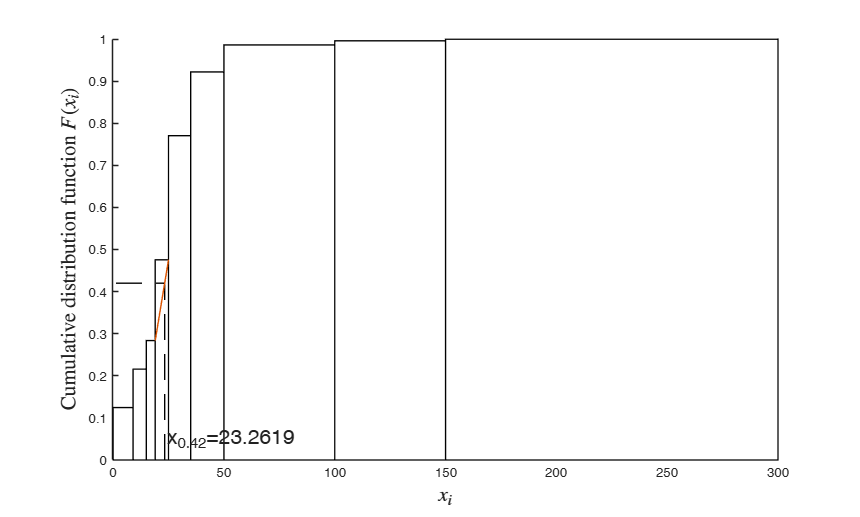GUIquantile

GUIquantile shows the necessary calculations to obtain $x_z$ quantile.

Syntax

• out=GUIquantile(x, z)example
• out=GUIquantile(x, z,Name,Value)example

Description

out =GUIquantile(x, z) Percentile "z" in an individual series.

out =GUIquantile(x, z, Name, Value) Computation of the median in presence of discrete data with frequencies.

Examples

expand all

Percentile "z" in an individual series.

In this case we find percentile 43, all frequencies are equal to 1.

x=[2 10 5 9 13];
GUIquantile(x,0.43)

Computation of the median in presence of discrete data with frequencies.

X = Number of components of Italian families (source ISTAT).

% freq = Number of families (in thousands).
x=1:6;
freq=[7910 6833 5116 4051 1088  303];
GUIquantile(x,0.5,'freq',freq,'DiscreteData',true)

Related Examples

expand all

Computation of the median in presence of discrete data with frequencies and related plot.

(See page 14 of [CMR]) X = 133 students grades on a given exam freq = frequencies.

x=18:30;
freq=[12 10 4 8 7 19 22 8 9 13 10 8 3 ];
% Find 83 percentile and show associated plot.
GUIquantile(x,0.83,'freq',freq,'DiscreteData',true,'plots',true)Example of computation of 40 per cent percentile in a frequency distribution (X is continuous).

% The following frequency distribution shows the amount (in thousands
% of Euros) of advertising expenditure made in a given month by a series of
% companies. (See page 15 of [CMR])
X=[9  1787
15  1310
19  972
25  2753
35  4227
50  2174
100 920
150  138
300  54
Inf  9];
x=[0;X(:,1)];
freq=X(:,2);
GUIquantile(x,0.42,'freq',freq,'DiscreteData',false,'plots',1)
ans =

11×5 table

i     x_{(i)}    n_{i}       f_i        F(x_i)
___    _______    _____    __________    _______

1        9       1787       0.12458    0.12458
2       15       1310      0.091327    0.21591
3       19        972      0.067764    0.28367
4       25       2753       0.19193     0.4756
5       35       4227       0.29469    0.77029
6       50       2174       0.15156    0.92185
7      100        920      0.064138    0.98599
8      150        138     0.0096207    0.99561
9      300         54     0.0037646    0.99937
10      Inf          9    0.00062744          1
NaN      NaN      14344             1        NaNInput Arguments

x — vector of numeric data. Vector.

Vector containing strictly numerical data.

Note that if x is referred to a continuous variable x(1) represent the lower extreme of the first class, and the other values of x contain the upper extremes of the other classes.

Data Types: double

z — requested probability. Scalar.

Requested probabilities for which to compute the quantile, specified as a scalar.

Data Types: double

Name-Value Pair Arguments

Specify optional comma-separated pairs of Name,Value arguments. Name is the argument name and Value is the corresponding value. Name must appear inside single quotes (' '). You can specify several name and value pair arguments in any order as Name1,Value1,...,NameN,ValueN.

Example: 'freq',1:10 , 'DiscreteData',false , 'plots',true

freq —frequencies.vector.

Vector of the same length of x containing the frequencies (weights) associated to each value of x if x is discrete or vector with length (x)-1 if variable X is continuous. If freq is not supplied, it is assumed that all observations have the same (relative) frequency (weight).

Example: 'freq',1:10

Data Types: double

DiscreteData —Discrete data or continuous data.boolean.

If DiscreteData is true (default), we assume that the data in x come from a discrete variable.

If DiscreteData is false, we assume that the data in x come from a continuous variable.

Example: 'DiscreteData',false

Data Types: boolean

plots —show quantile graphically.boolean.

If plots is true an additional plot which shows graphically how the quantile has been obtained using the linear interpolation is displayed on the screen. The default value of plots is false.

Example: 'plots',true

Data Types: boolean

Output Arguments

out —detailed output to compute the index. Table

Table with n+1 rows (where n is the length of x) containing what is shown in the GUI. Last row contains the totals.

Cerioli, A., Milioli, M.A., Riani, M. (2016), "Esercizi di statistica (Quinta edizione)". [CMR]